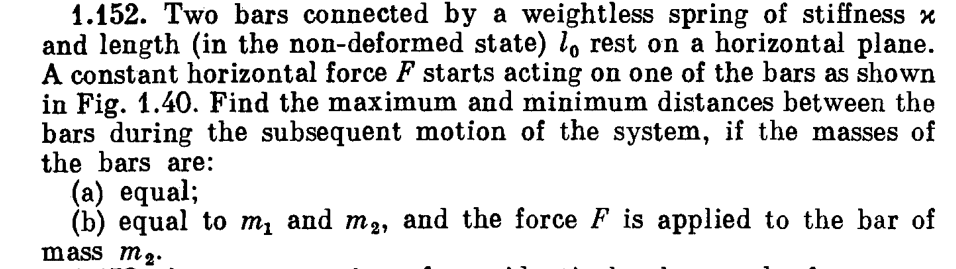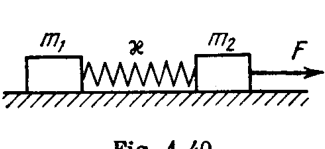# Easy problem about two bars connected by a spring

LCSphysicist
Homework Statement:
All below.
Relevant Equations:
All below.My solution:
The distance between the block is x2-x1.
x2'' = F/m2 - k(x2-x1)/m2
x1'' = k(x2-x1)/m1

x2''-x1'' = x''.

x'' = F/m2 - kx(1/m1 + 1/m2)

Being y = (1/m1 + 1/m2)

That is>

x = (lo - Fy/m2k)*cos(wt) + Fy/m2k

xmin = lo
xmáx = -lo + 2*F*y/(m2*k) = -lo + 2*m1*F/k(m1+m2)

But the answer to xmax is lo + 2*m1*F/k(m1+m2)

Do you see any error?

Homework Helper
Gold Member
2022 Award
The way you used x1, x2 and x, you effectively defined these as though l0=0. That's fine so long as you remember to add l0 back right at the end. But somehow you got it as being multiplied by the cos term.

•LCSphysicist
LCSphysicist
The way you used x1, x2 and x, you effectively defined these as though l0=0. That's fine so long as you remember to add l0 back right at the end. But somehow you got it as being multiplied by the cos term.

I am not sure if i understood, are you saying that maybe i made an error in resolving the differential equation?

x'' = F/m2 - kx(1/m1 + 1/m2)
x = Acos(wt) + Bsin(wt) + Fy/m2k (actually 1/y = 1/m1 + 1/m2)
xo = lo = A + Fy/m2k
x'o = vo = 0 = Bw
B equals zero
A equals lo - Fy/m2k

So we get the final answer
(lo - Fy/m2k)*cos(wt) + Fy/m2k
?

You use ##kx## for your force, but ##x## is the distance between the bars whilst ##x-l_0## is the extension! If you replace change your equations accordingly, you will get that the general solution is then $$x = A\cos{(\omega t)} + \frac{F}{kym_2} + l_0$$ and you can deduce that ##A = -\frac{F}{kym_2}## with ##x(0) = l_0##, which then gives you the correct result.

•LCSphysicist
LCSphysicist
Oh i betrayed myself>>>>>>>>>>>>>>>:O

thank you all.

Homework Helper
Gold Member
2022 Award
I am not sure if i understood, are you saying that maybe i made an error in resolving the differential equation?

x'' = F/m2 - kx(1/m1 + 1/m2)
x = Acos(wt) + Bsin(wt) + Fy/m2k `(actually 1/y = 1/m1 + 1/m2)
xo = lo = A + Fy/m2k
x'o = vo = 0 = Bw
B equals zero
A equals lo - Fy/m2k

So we get the final answer
(lo - Fy/m2k)*cos(wt) + Fy/m2k
?
I am saying that your solution was technically in error right at the start:
The distance between the block is x2-x1.
x2'' = F/m2 - k(x2-x1)/m2

If the distance between the blocks is x2-x1 then the tension is k(x2-x1-l0).
If you redefine your x variables so as to exclude l0 then all your equations work, but when you solve the ODE there will be no l0.
Having solved it, and obtained the min and max values of x, you can add l0 back to get the min and max values of the separation.

•LCSphysicist and etotheipi
I wonder how many other ways there are of doing it. One might be to consider that ##a_{CM} = \frac{F}{(m_1 + m_2)}##. If we then transform into the frame of the CM, then both masses are performing SHM under the action of the inertial forces ##-\frac{Fm_1}{(m_1 + m_2)}## and ##-\frac{Fm_2}{(m_1 + m_2)}## respectively.

Once you work out the initial ratio of the lengths of spring on either side of the CM in terms of ##l_0##, then you can also work out the effective spring constants in terms of ##k##. And it's just like two springs in a gravitational field! You can quickly calculate the equilibrium positions and from that obtain the final result.

Maybe it takes a little longer than your method in this case; I'll try it tomorrow!

•LCSphysicist